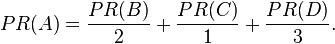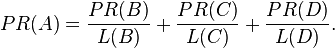### Algorithm Of PageRank

PageRank is a probability distribution used to represent the likelihood that a person randomly clicking on links will arrive at any particular page. PageRank can be calculated for collections of documents of any size. It is assumed in several research papers that the distribution is evenly divided between all documents in the collection at the beginning of the computational process. The PageRank computations require several passes, called "iterations", through the collection to adjust approximate PageRank values to more closely reflect the theoretical true value.

A probability is expressed as a numeric value between 0 and 1. A 0.5 probability is commonly expressed as a "50% chance" of something happening. Hence, a PageRank of 0.5 means there is a 50% chance that a person clicking on a random link will be directed to the document with the 0.5 PageRank.

Simplified Algorithm

Assume a small universe of four web pages: A, B, C and D. The initial approximation of PageRank would be evenly divided between these four documents. Hence, each document would begin with an estimated PageRank of 0.25.

In the original form of PageRank initial values were simply 1. This meant that the sum of all pages was the total number of pages on the web. Later versions of PageRank (see the below formulas) would assume a probability distribution between 0 and 1. Here we're going to simply use a probability distribution hence the initial value of 0.25.

If pages B, C, and D each only link to A, they would each confer 0.25 PageRank to A. All PageRank PR( ) in this simplistic system would thus gather to A because all links would be pointing to A.This is 0.75.

Again, suppose page B also has a link to page C, and page D has links to all three pages. The value of the link-votes is divided among all the outbound links on a page. Thus, page B gives a vote worth 0.125 to page A and a vote worth 0.125 to page C. Only one third of D's PageRank is counted for A's PageRank (approximately 0.083).In other words, the PageRank conferred by an outbound link L( ) is equal to the document's own PageRank score divided by the normalized number of outbound links (it is assumed that links to specific URLs only count once per document).In the general case, the PageRank value for any page u can be expressed as:i.e. the PageRank value for a page u is dependent on the PageRank values for each page v out of the set Bu (this set contains all pages linking to page u), divided by the number L(v) of links from page v.

Source: Wikipedia (under GNU license), updated as necessary by Isaac Yassar